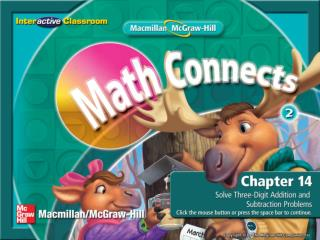DownloadDownload PresentationSplash Screen

# Splash Screen

Télécharger la présentation## Splash Screen

- - - - - - - - - - - - - - - - - - - - - - - - - - - E N D - - - - - - - - - - - - - - - - - - - - - - - - - - -
##### Presentation Transcript

1. Splash Screen

2. Are You Ready for Chapter 14? • Lesson 1: Add Hundreds • Lesson 2: Regroup Ones • Lesson 3: Regroup Tens • Lesson 4: Problem-Solving Strategy: Make a Table • Lesson 5: Estimate Sums • Lesson 6: Subtract Hundreds • Lesson 7: Regroup Tens • Lesson 8: Regroup Hundreds • Lesson 9: Estimate Differences • Lesson 10: Problem-Solving Investigation: Choose a Strategy • Chapter 14 Review Select a hyperlink to go to the corresponding slides. Chapter Menu

6. Introduction—End

7. I will add numbers in the hundreds. Lesson 1—Get Ready 1

9. 5 0 0 • How can you solve 500 + 400? 4 0 0 Lesson 1—Teach

10. 5 0 0 • What addition fact can you use to solve this problem? 4 0 0 5 + 4 = 9 Lesson 1—Teach

11. 5 0 0 • What is500 + 400? 4 0 0 9 0 0 900 Lesson 1—Teach

12. Lesson 1—Teach

13. Add. 300 + 200 = ___ 500 500 + 100 = ___ 600 700 + 200 = ___ 900 400 + 400 = ___ 800 Lesson 1—Check

14. Lesson 1—End

15. I will regroup the ones to add three-digit numbers. Lesson 2—Get Ready 1

16. regroup Lesson 2—Get Ready 2

17. 1 Lesson 2—Get Ready 3

20. How do you solve 611 + 319? 6 1 1 3 1 9 Lesson 2—Teach

21. Add the ones. What is 1 + 9? 6 1 1 3 1 9 10 Lesson 2—Teach

22. 1 • Regroup. 10 ones is the same as 1 ten. Where do we write the 1? 6 1 1 3 1 9 above the numbers in the tens column Lesson 2—Teach

23. 1 • What do we write in the ones place? 6 1 1 3 1 9 0 0 Lesson 2—Teach

24. 1 • Add the tens. What is 1 + 1 + 1? 6 1 1 3 1 9 3 0 3 Lesson 2—Teach

25. 1 • Add the hundreds. What is 6 + 3? 6 1 1 3 1 9 9 3 0 9 Lesson 2—Teach

26. 1 • What is 611 + 319? 6 1 1 3 1 9 9 3 0 930 Lesson 2—Teach

27. Add. 518 + 239 268 + 316 732 + 129 861 757 584 Lesson 2—Check

28. Lesson 2—End

29. I will regroup tens to add three-digit numbers. Lesson 3—Get Ready 1

33. How do you solve 399 + 148? 3 9 9 1 4 8 Lesson 3—Teach

34. Add the ones. What is 9 + 8? 3 9 9 1 4 8 17 Lesson 3—Teach

35. 1 • How do we regroup 17? 3 9 9 1 4 8 7 1 ten and 7 ones Lesson 3—Teach

36. 1 • Add the tens. What is1 + 9 + 4? 3 9 9 1 4 8 7 14 Lesson 3—Teach

37. 1 1 • How do we regroup 14 tens? 3 9 9 1 4 8 4 7 1 hundred and 4 tens Lesson 3—Teach

38. 1 1 • Add the hundreds. What is 1 + 3 + 1? 3 9 9 1 4 8 4 5 7 5 Lesson 3—Teach

39. 1 1 • What is399 + 148? 3 9 9 1 4 8 4 5 7 547 Lesson 3—Teach

40. Add. 482 + 255 284 + 243 182 + 226 408 737 527 Lesson 3—Check

41. Lesson 3—End

42. I will make a table to solve problems. Lesson 4—Get Ready 1

44. Lesson 4—Teach

45. What do I know? Underline what you know. Lesson 4—Teach

46. What do I need to find? Circle the question. Lesson 4—Teach

47. How will I solve the problem? I will make a table to find the time that Sonja will leave. Lesson 4—Teach

48. 2 4:30 3 6:30 Make a table. Sonja’s plane will leave at 6:30. Lesson 4—Teach

49. Look back.Is my answer reasonable? Flight 1 leaves at 2:30. Flight 2 leaves at 4:30. Flight 3 leaves at 6:30. yes Lesson 4—Teach

50. Make a table to solve. • Conner read 1 book every week for 6 weeks. Each book had 100 pages. How many pages did he read in all? 600 pages Lesson 4—Check